# Preparation Manual

## Section 4: Sample Selected-Response Questions TX PACT: Physical Science: Grades 6–12 (737)

This section presents some sample exam questions for you to review as part of your preparation for the exam. To demonstrate how each competency may be assessed, sample questions are accompanied by the competency that they measure. While studying, you may wish to read the competency before and after you consider each sample question. Please note that the competency statements do not appear on the actual exam.

The correct answer is provided for each sample exam question. The sample questions are included to illustrate the formats and types of questions you will see on the exam; however, your performance on the sample questions should not be viewed as a predictor of your performance on the actual exam.

The following reference materials will be available to you during the exam:

### Domain I—Nature of Science

#### Competency 001—Understand principles and procedures of scientific inquiry.

Data for the variables x and y are collected during an experiment and given in the table below.

 x y 5 10 15 20 25 30 0.05 0.19 0.32 0.47 0.61 0.75

1. Which of the following best describes the relationship between x and y?

1. constant
2. linear
3. inverse
Option B is correct. This question requires the examinee to analyze scientific data. The x-values of the data in the table increase in increments of 5, that is, Delta x equals 5. The y-values increase in increments of 0.14, 0.13, 0.15, 0.14, and 0.14, respectively. Hence, the ratiodelta y over delta x is approximately constant and the relationship is best described as being linear.

2. Which of the following is best accomplished using a mass spectrometer?

1. determining the percent abundance of an element's natural isotopes
2. determining the triple point of an unknown substance
3. determining the reaction rate for a chemical reaction involving a gas
4. determining the electronegativity value of an element
Option A is correct. This question requires the examinee to demonstrate knowledge of the equipment used in chemistry. A mass spectrometer measures the masses and relative abundance of atomic or molecular ions in a sample. These numerical values can be used to calculate the percent abundance of an element's natural isotopes.

#### Competency 002—Understand the history of science, its connections with other sciences, and the relationships among science, technology, and society.

3. Which of the following best describes how the invention of X-ray diffraction in physics contributed to the biological and chemical sciences?

1. by generating clear images of bacteria and viruses
2. by determining the structure of molecules
3. by discovering missing elements in the periodic table
4. by producing disease-resistant plant varieties
Option B is correct. This question requires the examinee to demonstrate knowledge of how science and technology affect each other. Because the wavelength of X-rays is of the same order as the size of molecules, X-ray diffraction allows scientists to infer structural properties of substances, such as DNA, by analyzing the patterns produced by the waves as they are diffracted from the substance.

DNA → RNA → protein

4. Which of the following biological processes does the pathway shown above summarize?

1. mitosis
2. cellular respiration
3. meiosis
4. gene expression
Option D is correct. This question requires the examinee to demonstrate knowledge of major contemporary concepts in biology. DNA is a molecule that stores genetic information in cells. The pathway shows the translation of genetic information from DNA to RNA to protein, a process that leads to the active expression of genetic information.

Flexible polyurethane foams are used in many commercial products, such as upholstered furniture, bedding, automobile seats, and sponges. An important component in the manufacturing of flexible polyurethane foams are polyols, which are typically derived from petroleum products. In 2007, Cargill, Incorporated, was awarded a Presidential Green Chemistry Challenge Award from the Environmental Protection Agency (EPA) for its research into polyols made from renewable biological resources. The quality and performance of the polyurethane foam made from these non-petroleum-based polyols proved comparable to the quality and performance of the polyurethane foam made from petroleum-based polyols.

5. The passage above illustrates which of the following about the relationships among science, technology, and society?

1. Private industry is reluctant to comply with EPA standards without strict government oversight.
2. Chemistry research can play a significant role in solving major environmental problems.
3. Environmentally friendly technologies can only be competitive with government support.
4. Applied chemistry research is increasing due to a decrease in public funding for basic research.
Option B is correct. This question requires the examinee to analyze the interrelationships among science, technology, and society. Knowledge of chemistry can be used to assess existing manufacturing protocols and to propose alternative procedures. These new procedures can play a significant role in mitigating the environmental problems facing society.

### Domain II—Mechanics

#### Competency 003—Understand motion in one and two dimensions.

6. A ball at rest on level ground is kicked into the air. The ball strikes the ground 4.00 s later at a horizontal distance of 40.0 m from where it was kicked. What is the magnitude of the initial velocity of the ball?

1. 10.0 m slash s
2. 19.6 m slash s
3. 22.0 m slash s
4. 40.5 m slash s
Option C is correct. This question requires the examinee to apply properties of vectors to analyze projectile motion. Since the ball travels a horizontal distance of 40.0 m in 4.00 s, the horizontal component of its initial velocity is 10.0 m slash s. The ball is in flight for a total of 4.00 s, so it reaches its maximum height in 2.00 s. Using the equation v left parenthesis t right parenthesis equals negative 9.8t plus v subscript 0 subscript y and v left parenthesis 2.00 right parenthesis equals 0.00 gives v subscript 0 subscript y equals 19.6 m slash s. The magnitude of the initial velocity is thereforev 0 equals square root left parenthesis 10.0 right parenthesis squared plus left parenthesis 19.6 right parenthesis squared equals 22.0 m slash s.

#### Competency 004—Understand forces as interactions and their effects on motion.

A rope with a tension of 10.0 N is attached to a 2.00 kg mass. The rope makes an angle of 30.0 degrees with the horizontal.There is a diagonal line labeled 10.0 N represents the rope and forms the top part of the angle. And a dotted horizontal line is shown as the base of the angle.

7. What is the normal force on the mass?

1. 5.0 N
2. 9.8 N
3. 14.6 N
4. 19.6 N
Option C is correct. This question requires the examinee to solve problems involving force and motion. There are three forces acting on the mass in the y-direction: the gravitational force, the normal force, and the vertical component of the tension in the string. The normal force is perpendicular to the surface of the mass and in the positive y-direction. From Newton's second law, sigma-summation F equals ma applied in the y-direction, sigma-summation F subscript y equals 0 equals negative mg plis N plus T sine 30 degrees. Using g equals 9.80 m slash s squared, substituting the values given, and solving for the normal force gives N equals 14.6 N.

#### Competency 005—Understand the conservation of energy and linear momentum.

A mass initially at rest at a height of 6r slides down a frictionless track and around a circular loop of radius r.The track is inclined at about 60 degrees that slopes down from a height dimensioned as 6r to the lower edge of a circular loop with a radius dimensioned as r. The track is tangent to the loop, and the top point of the loop is labeled A.

8. What is the speed of the mass at the top of the circular loop (point A)?

1.The square root of 4gr
2.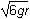The square root of 6gr
3.The square root of 7gr
4.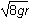The square root of 8gr
Option D is correct. This question requires the examinee to apply the work-energy theorem to conservative systems. Since there are no frictional forces, the work done by friction is zero and the total mechanical energy is conserved. Therefore, mg left parenthesis 6r right parenthesis equals 1 half mv squared plus mg left parenthesis 2r right parenthesis. Solving this equation for v gives v equals the square root of 8gr.

#### Competency 006—Understand simple harmonic motion and rotational dynamics.

A mass on a spring is displaced from equilibrium and released. The graph below shows the velocity of the mass versus time.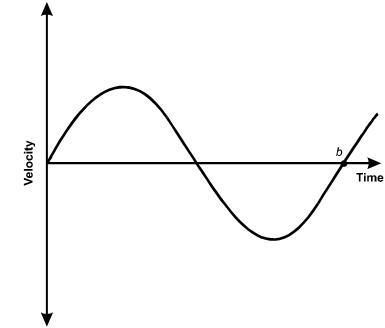A sinusoid line starts at the origin with an upward slope. After the upper curve and the lower curve, the line passes through the horizontal axis at point b.

9. Which statement about the mass at time b must be true?

1. It is located at its equilibrium position.
2. Its potential energy is equal to zero.
3. Its kinetic energy is at a maximum.
4. Its acceleration is at a maximum.
Option D is correct. This question requires the examinee to use graphs of trigonometric functions to analyze characteristics of simple harmonic motion. The graph shows the velocity of a mass oscillating on a spring. At point b the velocity is zero, so the mass is at one of its turning points and the displacement of the mass, x, is either A or negative A, where A is the amplitude of motion. Since the slope of the line tangent to the curve at b is positive, the acceleration is positive, so x equals negative A. Since F equals ma equals negative kx, the force, and therefore the acceleration, is maximum when x equals negative A.

### Domain III—Electricity and Magnetism

#### Competency 007—Understand properties of the electric field.

Three identical point charges are located on the vertices of an equilateral triangle of side length s.10. What is the vertical component of the electrostatic force on the charge located on vertex P?

1. kq squared over s squared sine 30 degrees
2. kq squared over s squared cosine 30 degrees
3. 2kq squared over s squared sine 30 degrees
4. 2kq squared over s squared cosine 30 degrees
Option D is correct. This question requires the examinee to describe an electric force for a simple charge distribution. From Coulomb's law, the magnitude of the force on q from the left-most charge on the base of the triangle is F equals fraction kqq over s squared, and the component in the vertical direction is kqq over s squared cosine theta, where theta equals 30 degrees. This force points in the positive y-direction. The same applies for the force from the right-most charge. The net force is the superposition of the two forces, which is F equals >2kq squared over s squared cosine 30 degrees.

#### Competency 008—Understand properties of the magnetic field and electromagnetic induction.

A wire that carries conventional current I in the direction shown is in a magnetic field that points into the plane of the page.11. Which arrow shows the direction of the force on the wire?

1.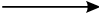2.3.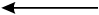4.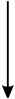Option C is correct. This question requires the examinee to analyze the magnetic force on a moving charge in a magnetic field. The diagram shows a magnetic field that points into the page. The wire carries conventional current, which implies positive charges moving toward the top of the page. Since start bold F end bold equals I samll l times start bold B end bold and small l represents the direction of the conventional current I, the right-hand rule can be used to cross start bold v end bold into start bold B end bold. This shows that the force on the wire points to the left, as shown in response C.

#### Competency 009—Understand properties of electric circuits.

12. A resistor is connected in series across the terminals of an ideal battery of voltage V. The resistor dissipates power W from the battery. A second, identical resistor is connected in series with the first resistor. What is the total power dissipated by the two resistors?

1. W over 2
2. W
3. 2W
4. 4W
Option A is correct. This question requires the examinee to analyze an electric circuit in terms of energy or power. The power dissipated by the resistor in the circuit is given by P equals IV equals W, where I is the current through the resistor. When a second resistor is added to the circuit, the effective resistance doubles. Since the voltage of the battery is the same, the current is 1 half I. Since there are two identical resistors in the circuit, the voltage across each is 1 half V and the power dissipated by each is P equals 1 fourth W. Therefore, the total power dissipated is P equals 1 half W.

### Domain IV—Waves

#### Competency 010—Understand the fundamental properties of waves.

13. A person standing 85 m from a wall shouts and hears the echo 0.50 s later. The person then plays a note of 440 Hz on a flute. What is the wavelength of the note in air?

1. 0.39 m
2. 0.77 m
3. 1.3 m
4. 2.6 m
Option B is correct. This question requires the examinee to solve a problem involving wave speed, frequency, and wavelength. Since the person hears the echo of the shout after 0.50 s, the speed of sound is 170 m slash 0.50 s or 340 m slash s. Since v equals f lambda, the wavelength is lambda equals 0.77 m.

#### Competency 011—Understand the characteristics of light and electromagnetic radiation.

14. A light-emitting diode (LED) on a consumer device emits 0.020 W of light of wavelength lambda equals 650 times 10 superscript negative 9 m. Which of the following expressions equals the number of photons emitted by the LED per second in terms of Planck's constant (h) and the speed of light (c)?

1. 0.020 lambda over hc

2. 0.020hcover lambda

3. 0.020h lambda over c

4. 0.020 over hc lambda
Option A is correct. This question requires the examinee to describe various phenomena using the photon model of light. The energy of a single photon is given by E equals hf. For light, c equals f lambda, so E equals hc over lambda;. Since the power of the LED is 0.020 W, dividing the power by the energy of a single photon gives the number of photons emitted per second.

### Domain V—Modern Physics

#### Competency 012—Understand thermal energy and the kinetic theory of matter.

A closed, insulated vessel consists of a paddle wheel immersed in 0.50 kg of water at room temperature. The shaft of the paddle wheel is attached to a motor that has an output of 25 W. The paddle wheel rotates, churning the water.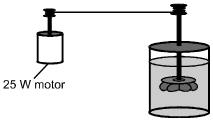15. Disregarding the heat capacity of the vessel and paddle, what is the change in temperature of the water if the motor is run for 5.0 minutes?

1. 0.03 degrees Celsius
2. 1.0 degrees Celsius
3. 1.8 degrees Celsius
4. 3.6 degrees Celsius
Option D is correct. This question requires the examinee to solve a problem related to specific heat and the first law of thermodynamics. The first law of thermodynamics states that Delta E equals Delta Q minus W, where W is the work done by the system. Since the vessel is insulated, Delta Q equals 0. Therefore, Delta E equals W, since work is done on the system as the paddle wheel churns the water. Therefore, c subscript w msubscript w Delta T equals left parenthesis 25 W right parenthesis left parenthesis 5 min right parenthesis left parenthesis 60 s slash min right parenthesis. Using the value c subscript w equals 4.19 times 10 cubed J slash left parenthesis kg dot degrees Celsius right parenthesis given in the Constants and Formulas and solving gives Delta T equals 3.6 degrees Celsius.

#### Competency 013—Understand fundamental ideas of modern physics.

Electrons from an electron gun pass through a double slit before striking a screen. A counter records the number of electrons that arrive at the screen as a function of x, the position along the screen. The distribution of the number of electrons arriving is shown below.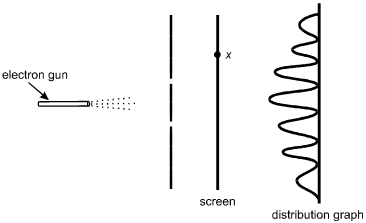The electron gun is on the left, pointed horizontally to the right. Dotted lines represent the electrons spraying toward the right. A vertical line with two breaks, located symmetrically above and below the level of the electron gun, is between the gun and a vertical line representing the screen. A point on the screen is labeled x, somewhat above the level of the upper slit. To the right of the screen is a distribution graph with an oscillating sinusoidal wave on a vertical baseline. The peaks are to the left of the baseline. The highest peak is in the center, at the level of the electron gun, with peaks progressively lower at the upper and lower ends of the graph.

16. According to the principles of quantum mechanics, the distribution pattern shown is a result of:

1. electrons interfering due to their wave properties.
2. electrons striking the edges of the slits while passing through.
3. photons interacting with each other on either side of the slits.
4. electrons interacting with photons prior to arriving at the screen.
Option A is correct. This question requires the examinee to demonstrate knowledge of the wave-particle duality. In the double-slit experiment shown, the distribution of the number of electrons arriving on the screen at various positions is a result of interference. Interference is a consequence of the wave properties of electrons.

#### Competency 014—Understand the fundamental principles of nuclear physics.

17. If superscript A over subscript Z base X represents an atomic nucleus prior to gamma decay, which of the following represents the nucleus after gamma decay?

1. superscript A over subscript Z base X

2. superscript A negative 1 over subscript Z base X

3. superscript A negative 1 over subscript Z base X

4. superscript A negative 4 over subscript Z negative 2 base X
Option A is correct. This question requires the examinee to apply knowledge of radioactive decay processes. Gamma rays are electromagnetic waves, or photons, emitted as the nucleus of an atom transitions from a higher energy state to a lower energy state. Hence, the number and type of nucleons in the nucleus does not change.

### Domain VI—Matter and Atomic Structure

#### Competency 015—Understand the properties of matter.

18. Which of the following only occurs during a nuclear change?

1. Valence electrons are raised to higher energy levels.
2. Two or more types of atoms are combined.
3. Energy is released to the surroundings.
4. An element's atomic number is reduced.
Option D is correct. This question requires the examinee to demonstrate knowledge of the characteristics of radioactive materials. Chemical and physical changes do not involve changes within the nucleus of an atom and therefore would not lead to a reduction in an element's atomic number. This type of change would only result from a nuclear change.

#### Competency 016—Understand atomic theory and the periodic table.

19. Which of the following elements is the most electronegative?

1. hydrogen
2. fluorine
4. francium
Option B is correct. This question requires the examinee to demonstrate knowledge of the periodic table and its usefulness in predicting the relative reactivity of given elements. The electronegativity of elements in the periodic table tends to increase from bottom to top within a group and from left to right across a period. Of the given elements, fluorine is in the uppermost position on the right-hand side of the periodic table.

#### Competency 017—Understand the kinetic molecular theory, the nature of phase changes, and the gas laws.

20. A gas occupies a volume of 1.25 liters at a pressure of 825 mm Hg. What will be the final pressure of this gas if it is compressed into a volume of 725 mL at constant temperature?

1. 479 mm Hg
2. 748 mm Hg
3. 1.30 times 10 cubed mm Hg
4. 1.42 times 10 cubed mm Hg
Option D is correct. This question requires the examinee to solve a problem involving the gas laws. If the temperature and number of moles of a gas are held constant, the relationship between initial pressure and initial volume and a new pressure and a new volume is P subscript 1 V subscript 1 equals P subscript 2 V subscript 2. This relationship can be used to calculate the new pressure when the initial pressure, initial volume, and new volume are known.

### Domain VII—Energy and Chemical Bonding

#### Competency 018—Understand the principles of thermodynamics and calorimetry.

21. A 4.75 g sample of solid NaOH is dissolved in 50.5 g of H2O in a constant-pressure calorimeter having a heat capacity of 18.5 joules per degrees Celsius. The temperature rises from 21.1 degrees Celsius to 33.6 degrees Celsius. Assuming that the solution has a specific heat capacity of 4.184 joules per gram per degrees Celsius and negligible heat loss from the calorimeter, how much heat is produced in the solution process?

1. 2.64 kJ
2. 2.89 kJ
3. 3.12 kJ
4. 4.27 kJ
Option C is correct. This question requires the examinee to analyze the results of a calorimetry experiment. The total heat produced in the given solution process is equal to the heat absorbed by the solution plus the heat absorbed by the calorimeter. The heat absorbed by the solution is calculated using the equation q equals m times s times Delta T. The heat absorbed by the calorimeter is equal to C subscript calorimeter times Delta T.

#### Competency 019—Understand energy relationships in chemical bonding, chemical reactions, and physical processes.

Bond Bond Enthalpy
(kJ/mol)
H—H 436.4
H—Cl 431.9

H2(g) plus Cl2(g) right arrow 2HCl(g) new line Delta H degrees subscript rxn equals negative 184.6 kJ

22. Given the information shown above, what is the best estimate of the bond enthalpy for the Cl—Cl bond?

1. 189.1 kJ/mol
2. 242.8 kJ/mol
3. 256.3 kJ/mol
4. 585.1 kJ/mol
Option B is correct. This question requires the examinee to analyze energy changes due to the formation or breaking of chemical bonds. The enthalpy change for a chemical reaction is equal to the sum of the enthalpy changes involved in breaking existing bonds minus the sum of the enthalpy changes involved in forming new bonds. The bond enthalpy for the Cl—Cl bond can be calculated using this relationship and the given bond enthalpies for H—H and H—Cl.

#### Competency 020—Understand the nomenclature and structure of inorganic and organic compounds.

23. Which of the following structural formulas represents 4–ethyl-3, 3–dimethylhexane?

1.2.3.4.Option C is correct. This question requires the examinee to apply the International Union of Pure and Applied Chemistry (IUPAC) rules of nomenclature. 4-ethyl-3, 3-dimethylhexane is an alkane consisting of six continuous carbon atoms. An ethyl group (C2H5) is attached to the number 4 carbon and two methyl groups (CH3) are attached to the number 3 carbon.

#### Competency 021—Understand chemical bonding and intermolecular forces and their effect on the properties of substances.

24. The high melting point of diamond is due to:

1. strong covalent bonds between carbon atoms.
2. an irregular, three-dimensional crystal structure.
3. delocalized, highly mobile bonding electrons.
4. extensive van der Waals forces between carbon atoms.
Option A is correct. This question requires the examinee to relate the properties of substances to their atomic bonds. Diamond is a covalent-network crystalline solid. The carbon atoms in this network are linked by covalent bonds. This strong bonding between carbon atoms is responsible for the high melting point of diamond.

### Domain VIII—Chemical Reactions

#### Competency 022—Understand the nature of chemical reactions.

25. Which of the following products is formed by an esterification reaction between acetic acid (CH3CO2H) and ethanol (CH3CH2OH)?

1.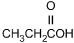2.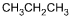3.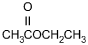4.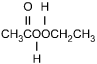Option C is correct. This question requires the examinee to analyze different types of chemical reactions. In the esterification reaction between acetic acid and ethanol, the O H group from acetic acid and the H attached to the O in ethanol combine to form water. The remaining portions of the acetic acid and ethanol molecules combine to form the ester, ethyl acetate.

#### Competency 023—Understand the principles of chemical equilibrium.

Zn left parenthesis s right parenthesis plus 2H positive left parenthesis aq right parenthesis equilibrium arrow Zn superscript 2 positive left parenthesis aq right parenthesis plus H2 left parenthesis g right parenthesis

26. Which of the following is the equilibrium constant expression for the equation shown above?

1.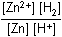2.3.4.Option D is correct. This question requires the examinee to solve problems involving equilibrium constants. When writing equilibrium constant expressions, pure solid and pure liquid compounds are omitted and the pressure of gaseous compounds can be used in place of concentration. The equilibrium constant expression for this reaction is equal to the concentration of Zn superscript 2 positive left parenthesis aq right parenthesis times the pressure of H2 left parenthesis g right parenthesis each raised to a power equal to its stoichiometric coefficient, divided by the concentration of H superscript positive left parenthesis aq right parenthesis raised to a power equal to its stoichiometric coefficient.

#### Competency 024—Understand acid-base chemistry.

27. Which of the following explains why nitric acid (HNO3) is a stronger acid than nitrous acid (HNO2)?

1. The additional oxygen present in nitric acid increases the polarity of the O—H bond.
2. The extent of ionization is directly related to molecular weight when comparing related compounds.
3. The anion formed by removing H positive from nitrous acid is more stable than the anion formed by removing H positive from nitric acid.
4. The O—H bond in nitrous acid is weaker than the O—H bond in nitric acid.
Option A is correct. This question requires the examinee to demonstrate knowledge of the relationship between molecular structure and acid strength. The strength of an acid is a function of its tendency to ionize. For oxoacids with the same central atom, acid strength increases as the oxidation number of the central atom increases because of the resulting increase in polarity of the O—H bond. The oxidation number of nitrogen in HNO3 is positive 5 and in HNO2 it is positive 3, thus the O—H bond in HNO3 is more polar and ionizes more readily.

#### Competency 025—Understand oxidation-reduction reactions and electrochemistry.

28. Automobile mechanics measure the density of the electrolyte solution of lead storage batteries to determine the amount of charge remaining. Which of the following statements describes the cause of the change in electrolyte density as the battery's charge decreases?

1. The sulfuric acid electrolyte is consumed and water is formed.
2. Water evaporates and the electrolyte concentration increases.
3. The lead and lead left parenthesis IV right parenthesis oxide migrate from the solution to the electrodes.
4. Lead in the electrolyte solution precipitates out of the solution.
Option A is correct. This question requires the examinee to demonstrate knowledge of the applications of electrochemistry. During the normal operation of a lead storage battery, sulfuric acid is consumed and water is produced. The density of the electrolyte solution is related to how much of each of these substances is present in the solution.

### Domain IX—Stoichiometry and Solutions

#### Competency 026—Understand the mole concept.

29. Which of the following is equivalent to 1.42 times 10 to the power of 23 atoms?

1. 25.0 g Br
2. 15.0 g Cu
3. 19.0 g Sn
4. 23.0 g Mn
Option B is correct. This question requires the examinee to demonstrate knowledge of the mole concept. The number of moles of an element is equal to the product of the mass of the element divided by the molar mass of the element times Avogadro's number.

#### Competency 027—Understand stoichiometry.

N2 left parenthesis g right parenthesis plus 3H2 left parenthesis g right parenthesis right arrow 2NH3 left parenthesis g right parenthesis

30. Given the equation shown above, how much NH3 is formed when 823 g of N2 are combined with 145 g of H2?

1. 58.8 g
2. 96.7 g
3. 815 g
4. 1650 g
Option C is correct. This question requires the examinee to solve stoichiometric problems involving mass. By calculating the number of moles of each reactant and analyzing the stoichiometric relationship between them, H2 can be identified as the limiting reactant. From the stoichiometric relationship between H2 and NH3, the number of moles of product formed can be calculated. This mole quantity can then be converted into mass using the molar mass of NH3.

#### Competency 028—Understand the properties of solutions and colloidal suspensions.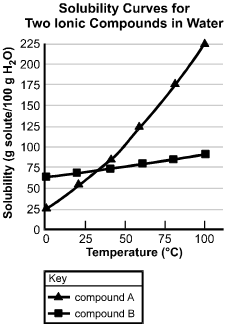The horizontal axis is labeled Temperature in degrees centigrade, and has values marked from 0 to 100 in increments of 25. The vertical axis is labeled "Solubility (g solute/100 g H sub 2 O)", and has values marked from 0 to 225 in increments of 25. The curve for compound A passes through the following data points (values are approximate): (0, 25); (20, 55); (45, 80); (55, 125); (80, 175); and (100, 225). The curve for compound B passes through the following data points (values are approximate): (0, 65); (20, 70); (45, 75); (57, 77); (80, 80); and (100, 90).

31. Based on the solubility curves shown above, which of the following procedures will be most effective in isolating the greatest amount of pure compound A from a mixture consisting of 200 g of compound A and 15 g of compound B?

1. dissolving the mixture in 100 g of water and then heating to the solution's boiling point
2. dissolving the mixture in 100 g of water at 100 degrees Celsius and then decreasing the temperature to 0 degrees Celsius
3. dissolving the mixture in 100 g of water at 75 degrees Celsius, filtering the solution, and then retaining the filtrate
4. dissolving the mixture in 100 g of water and then slowly increasing the temperature to 100 degrees Celsius